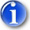Creating Cube FormulasYou have seen how creating Dimension hierarchies, and assigning Aggregate weights, results in the natural “summing up” of values. Cube formulas represent a powerful extension of your ability to perform mathematical calculations throughout a PowerOLAP database. With Cube formulas, you can perform all manner of calculations to populate a cell, ranges of cells, even entirely different Cubes.

Presently your Current Year Budget cube contains data for January for all Accounts and Regions. The following Cube formula will populate the month February.

1.   From the PowerOLAP ribbon, go to Model tab then select Cube command. The Cubes dialog box is opened

2.   Click on the Current Year Budget cube to select it.

3.   Click on the Formulas button. The Formula dialog box is opened.

We will now make use of some buttons in the Formula dialog box (also known as the ‘Formula Editor’). These buttons will enable us to specify the area of the Cube we want to populate with data, and where the data will come from.

4.   Click on the “squiggly brackets” or the Build Range Reference button,.
The Build Range Reference dialog box appears.

5.   For the Qualifier (top left), select the radio button Details.
This indicates that only Detail members are to be calculated by the Cube formula—Aggregate members will be calculated according to the Dimension hierarchy. (Note: it is possible to “overwrite” Hierarchy calculations via a Cube formula, a very important feature if you wish to calculate a “what if” or budgeted Aggregate data point, so that it contrasts to actual figures in Detail data points.)

6.   Select Months from the Dimension list then from the Members list box on the right, select February.

The Selected radio button is selected, as a consequence
The dialog box appears as follows, with the formula as it exists so far, at the bottom:7.   Click OK.

8.   Click on the “Equals“,,  button in the Formula Editor.
The left-hand side of the formula is completed, and is shown in the content area.9.   Next click on the “square brackets or the Build Cube Reference button,.
The Build Cube Reference dialog box appears.

10. Select the Current Year Budget from the cube drop down.

11. Again, select Months in the Dimension list and then select January from the Member list. The Selected radio button is selected, as a consequence.

Note that at the top of the dialog box, there is a Cubes drop-down. This brings up an important feature—the ability to create cross-cube formulas, which is explained in the PowerOLAP User Manual. There is only one Cube in our database, Current Year Budget, in the formula we are creating; data will come from this Cube, to populate another area of the same Cube.

The Build Cube Reference dialog box appears as follows:12. Click OK.
This return you to the Formula Editor (you can hit Enter after the “=” to show the formula on two lines)”13. Use the buttons in the Formula Editor — the asterisk (for multiplication), the numbers and the semi-colon — to complete the formula so that it appears like so:

Note: do not forget to put semi-colon (;) at the end of each formula statement.Following is a breakdown of the syntax of the Cube formula:

 DESCRIPTION Left of Equal ‘=’ Area of Cube to populate RHS Formula {“Months.February”} Dimension and Member to populate “Current Year Budget” Source cube [“Months.January”] Range within Source cube 1.5 Value (in this case: +50%) ; Ends formula statement

14. Click OK in the Formula Editor to save the formula then OK in the Cubes dialog (If you have mistyped the formula, you will receive a message indicating that there is syntax problem).

15. Back in the PowerOLAP main application window, go to Slice tab and click Recalculate command or simply press F9 in the Accounts by Months slice to recalculate values.

Notice that the February column has been populated by the Cube formula defined in the previous steps.Next, you will create a Cube formula that calculates a ratio of two Members. You will first need to add a new Member—Margin %—to the Accounts dimension, and then modify the Accounts dimension hierarchy. This Cube formula exercise brings up two important strengths of PowerOLAP, in comparison to static modeling tools, OLAP or otherwise: the capability to create new, “on-the-fly” calculations (which can of course be subsequently saved) for precisely specified (even new) components of a business model, which themselves are created entirely within PowerOLAP [i.e., not dependent on any static model of business data].

16. From the PowerOLAP ribbon, go to Model tab and select Dimension command.

17. Double click Accounts in the Dimension list box.
The ‘Accounts’ Hierarchy dialog box is displayed.

18. Click on the Create New Member button,, on the toolbar and type Margin % so that it appears in the Members list box.

Next step is to modify the Accounts dimension hierarchy.

19. Expand Accounts in the Hierarchy Definition box, on the right.

20. Select Margin % from the Members list box and drag it to the Hierarchy list box and release it just under Accounts.

The completed Hierarchy will appear as in the following figure:21. Click the OK buttonto close the Dimension Hierarchy dialog box.

22. Click OK in the Dimensions dialog box.
Margin %
now appears as the top row in the Accounts by Months slice.

Next, you will define a Cube formula that creates values for the new Margin %: the values will be based on a formula that divides Gross Profit by Net Sales:

23. From the PowerOLAP ribbon go to Model tab and select the Cube command. The Cubes dialog box appears.

24. Double-click the Current Year Budget cube to launch the Formula Editor.

25. Press Enter twice in the Content Area to move the previously written formula down two lines. Begin on the top line of the Formula Content area,
i.e., place this formula above the other
.ImportantPriority, which is top-to-bottom in the Formula editor, is very important for determining data calculations—consult the PowerOLAP® User Manual.

26. Using the Formula Editor dialog box, create the formula:

 All and {“Accounts.Margin %”}= “Current Year Budget”.[“Accounts.Gross Profit”] / “Current Year Budget”.[“Accounts.Net Sales”]*100;

27. Click OK in the Formula Editor then OK in the Cubes dialog.

28. Press F9 to recalculate values in the Accounts by Months slice.Margin % is now calculated for all Months in the Current Year Budget cube. The figure above shows the Margin % figures for United States. You can select other Regions members to verify that all Members in the Regions dimension have been updated as well.

Note though in the above slice that since we only have Net Sales and Cost of Sales data for January and February, hence, the rest of the months have zero Margin % values.

29. Click the File icon then Save Database command to save the data and slice (which now includes Margin %).